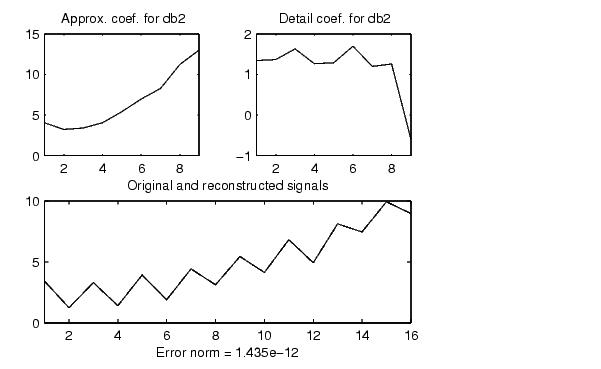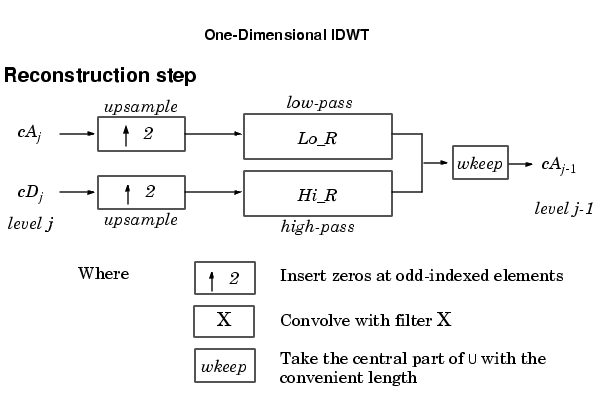Wavelet Toolboxidwt

Single-level inverse discrete 1-D wavelet transform

Syntax

• X = idwt(cA,cD,'wname')
X = idwt(cA,cD,Lo_R,Hi_R)
X = idwt(cA,cD,'wname',L)
X = idwt(cA,cD,Lo_R,Hi_R,L)
X = idwt(...,'mode',MODE)

Description

The idwt command performs a single-level one-dimensional wavelet reconstruction with respect to either a particular wavelet ('wname', see wfilters for more information) or particular wavelet reconstruction filters (Lo_R and Hi_R) that you specify.

X = idwt(cA,cD,'wname') returns the single-level reconstructed approximation coefficients vector X based on approximation and detail coefficients vectors cA and cD, and using the wavelet 'wname'.

X = idwt(cA,cD,Lo_R,Hi_R) reconstructs as above using filters that you specify.

• Lo_R is the reconstruction low-pass filter.
• Hi_R is the reconstruction high-pass filter.

Lo_R and Hi_R must be the same length.

Let la be the length of cA (which also equals the length of cD) and lf the length of the filters Lo_R and Hi_R; then length(X) = LX where LX = 2*la if the DWT extension mode set to periodization. For the other extension modes LX = 2*la-lf+2.

X = idwt(cA,cD,'wname',L) or X = idwt(cA,cD,Lo_R,Hi_R,L) returns the length-L central portion of the result obtained using idwt(cA,cD,'wname'). must be less than LX.

X = idwt(...,'mode',MODE) computes the wavelet reconstruction using the specified extension mode MODE.

X = idwt(cA,[],...) returns the single-level reconstructed approximation coefficients vector X based on approximation coefficients vector cA.

X = idwt([],cD,...) returns the single-level reconstructed detail coefficients vector X based on detail coefficients vector cD.

idwt is the inverse function of dwt in the sense that the abstract statement idwt(dwt(X,'wname'),'wname') would give back X.

Examples

• % The current extension mode is zero-padding (see dwtmode).

% Construct elementary one-dimensional signal s.

randn('seed',531316785)
s = 2 + kron(ones(1,8),[1 -1]) + ...
((1:16).^2)/32 + 0.2*randn(1,16);

% Perform single-level dwt of s using db2.

[ca1,cd1] = dwt(s,'db2');
subplot(221); plot(ca1);
title('Approx. coef. for db2');
subplot(222); plot(cd1);
title('Detail coef. for db2');

% Perform single-level inverse discrete wavelet transform,
% illustrating that idwt is the inverse function of dwt.

ss = idwt(ca1,cd1,'db2');
err = norm(s-ss);     % Check reconstruction.
subplot(212); plot([s;ss]');
title('Original and reconstructed signals');
xlabel(['Error norm = ',num2str(err)])

% For a given wavelet, compute the two associated
% reconstruction filters and inverse transform using
% the filters directly.

[Lo_R,Hi_R] = wfilters('db2','r');
ss = idwt(ca1,cd1,Lo_R,Hi_R);

% Using some plotting commands,
% the following figure is generated.Algorithm

Starting from the approximation and detail coefficients at level j, cAj and cDj, the inverse discrete wavelet transform reconstructs cAj-1, inverting the decomposition step by inserting zeros and convolving the results with the reconstruction filters.get idwt2